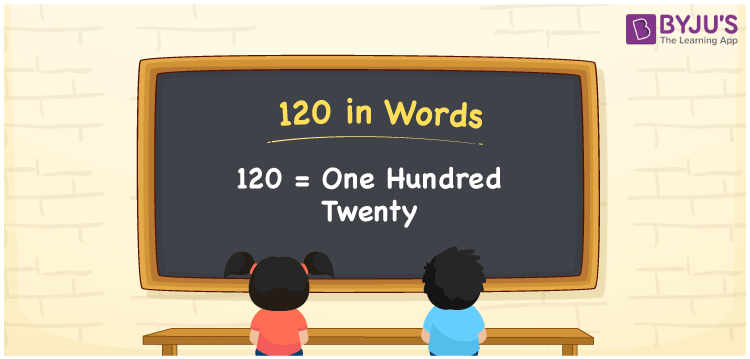# 120 in Words

120 in words is written as “One hundred twenty”. If you give 120 rupees to your friend, then you can say “I have given Rupees One hundred twenty to my friend”. In Mathematics, 120 is a natural number or an integer that is used to represent a value or a quantity. Learn to write the number 120 in words using the place value method and more about Numbers In Words at BYJU’S.

 120 in Words One hundred twenty One hundred twenty in numerical form 120

## 120 in English Words

120 in Words using English alphabets is expressed as given below:## How to Write 120 in Words?

120 in words is written based on the position of each digit in the given number. Since 120 is a three-digit number, thus the digits can be represented as:

 Hundreds Tens Ones 1 2 0

From the above table, we can see,

• 1 is at Hundreds
• 2 is at Tens
• 0 is at Ones

We need to read the number from right to left, in the table. Hence, it is spelt as One hundred twenty.

### Expanded Form of 120

We can write the expanded form of 120 as:

1 at Hundreds + 2 at Tens + 0 at Ones

= 1 × 100 + 2 × 10 + 0 × 1

= 100 + 20 + 0

= 120

= One hundred twenty

120 is a whole number that is succeeded by 119 and preceded by 121. Find more about the number 120 below:

• 120 in Words – One hundred twenty
• Is 120 an odd number? – No
• Is 120 an even number? – Yes
• Is 120 a perfect square number? – No
• Is 120 a perfect cube number? – No
• Is 120 a prime number? – No
• Is 120 a composite number? – Yes

## Frequently Asked Questions on 120 in words

Q1

### What is 120 in words?

120 in words is given by One hundred twenty.
Q2

### What is the rule to write 120 in the form of words?

120 in words is written based on the place values of each digit.

1 → Hundreds

2 → Tens

0 → Ones

Thus, the number is read as One hundred twenty.

Q3

### How to spell 120 in English words?

120 in English is spelt as One hundred twenty.
Test your Knowledge on 120 in Words